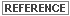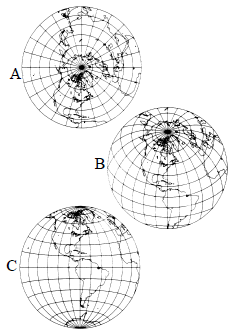Lambert Azimuthal Equal Area

Producer Field Guide

HGD_Product
Producer Field Guide
HGD_Portfolio_Suite
Producer

Lambert Azimuthal Equal Area projection is mathematically based on a plane tangent to the Earth. It is the only projection that can accurately represent both area and true direction from the center of the projection. This central point can be located anywhere. Concentric circles are closer together toward the edge of the map, and the scale distorts accordingly. This projection is well-suited to square or round land masses. This projection generally represents only one hemisphere.

 Construction Plane Property Equal-area Meridians Polar aspect: the meridians are straight lines radiating from the point of tangency. Oblique and equatorial aspects: meridians are complex curves concave toward a straight central meridian, except the outer meridian of a hemisphere, which is a circle. Parallels Polar aspect: parallels are concentric circles. Oblique and equatorial aspects: the parallels are complex curves. The Equator on the equatorial aspect is a straight line. Graticule spacing Polar aspect: the meridian spacing is equal and increases, and the parallel spacing is unequal and decreases toward the periphery of the projection. The graticule spacing, in all aspects, retains the property of equivalence of area. Linear scale Linear scale is better than most azimuthals, but not as good as the equidistant. Angular deformation increases toward the periphery of the projection. Scale decreases radially toward the periphery of the map projection. Scale increases perpendicular to the radii toward the periphery. Uses USGS uses in National Atlas (polar aspect).USGS uses in Circum-Pacific Map (polar, oblique, and equatorial aspects).

In the polar aspect, latitude rings decrease their intervals from the center outwards. In the equatorial aspect, parallels are curves flattened in the middle. Meridians are also curved, except for the central meridian, and spacing decreases toward the edges.

This projection contains these unique parameters:

Calculation Method    Select a method by which the transform is calculated.

Unspecified    Model used is unknown or not specified.

Sphere Formulas    Calculation is based on the model of the Earth as a sphere. This option is less accurate since the Earth is an ellipsoid, not a sphere; however it may be satisfactory for some maps.

Ellipsoid Formulas     Calculation is based on the model of the Earth as an ellipsoid. This option provides the more accurate reprojection within IMAGINE.See Spheroids for more information.

In Figure 122, three views of the Lambert Azimuthal Equal Area projection are shown:

A) Polar aspect, showing one hemisphere

B) Equatorial aspect, frequently used in old atlases for maps of the eastern and western hemispheres.

C) Oblique aspect, centered on 40°N.

Lambert Azimuthal Equal Area Projection